# Math Helper - Algebra Calculus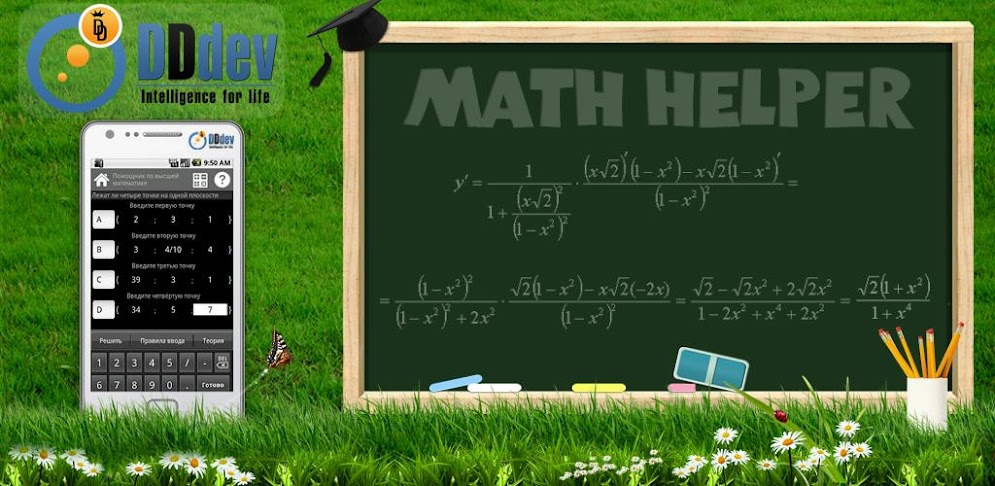## Description

Math Helper is the best application on the market, which solves mathematical problems and shows step by step solution.

It's easy - you enter mathematical problems and get the answer, detailed solution and a theory reference.

THIS IS ORIGINAL MATH HELPER. Avoid cheap clones of this app on the market and see no further – download it right now!

***
OUR USERS SAY:
«Excelent and helpful. Easy to use and very descriptive» - Julio Z.
«This is amazing app this helped me to study easily without any doubt.. Without any guidence i can get full marks in maths ... Love this app :) :) :D» - Pulkit G.
«This app helped me with my academics! Brought up my grades!» - Jordan P.

***
WHAT IT HAS
[✔] Linear Algebra - Operations with matrices (solving matrix)
[✔] Linear Algebra - Solving systems of linear equation (from 2 to 10 equation at a time)
[✔] Vector Algebra - Vectors (vectors problems)
[✔] Vector Algebra - Geometry Shapes (triangles, pyramids, planes)
[✔] Calculus - Derivative (Derivative of the function, of a function defined parametrically, of an implicit one)
[✔] Calculus - Indefinite Integrals (integrals solver, antiderivative)
[✔] Limits (limits calculator solver)
[✔] The theory of probability (probability theory, mathematical statistics)
[✔] Calculus - The number and sequence (prime factorization, lcm and gcd, complex numbers, arithmetic and geometric progressions and numbers, Diophantine equation, Euler function)
[✔] Function plotter (interactive graph plotter)
[✔] Also contains great scientific calculator and theoretical reference handbook
Math Helper is an universal assistant app for step by step solving mathematical problems for Algebra 1-2, Calculus (integral, Derivative, antiderivative, vectors, matrices (matrix), limits, equation, shapes, number etc) for school, secondary, college and university students and everyone who learn.
It allows you not only to see the answer or result of a task, but also a detailed step by step solution.
Also great feature is built-in scientific calculator and handbook – much easier to learn.

***
FEATURES AND ACHIEVEMENTS
✧ 14 topics and 80+ sub-section.
✧ Localization for Russian, English, Italian, Spanish, French, German and Portuguese
✧ Intel ® Learn Series Alliance quality mark
✧ EAS ® approved
✧ More than 30'000 customers all over the world supported development of our apps by doing purchase
✧ The application is equipped with a convenient multi function calculator and extensive theoretical reference handbook to learn better
Derivative, antiderivative, limits, geometric shapes, the task of statistics, probability theory, matrices or matrix (large spectrum of tasks with matrices (matrix) supported), systems of equation, finding probability, vectors and other mathematical solving – this and more in Math Helper!

***
SUPPORT
✪ Thank you all helping us to reach 2'500'000+ downloads of Lite version
✪ You could also support us with good feedback at Google Play or by links below (fb, twitter, email etc.)

***
WHAT IS NEXT
We have plans to implement
● Numbers and polynomial solving, division and multiplication
● Double integrals (antiderivative)
● More tasks on geometry, geometry plotter
● Limits step by step
● New applications, like Calculus symbolic calculator, Physics Solver, Physics Ref, Chemistry Solver, Geometry Shapes Solver and Plotter, theoretical reference handbook app.
***
Having problems with vectors, Probability theory, geometry shapes solution, integrals, matrices (matrix), graph plotter? Need an extensive theoretical reference handbook? Want to learn better? See no further – download this application right now!
Good for SSC, SAT, ACT or GCSE

P.s. Support us by liking us on Facebook

****** THANK YOU!!! ******

## Screenshots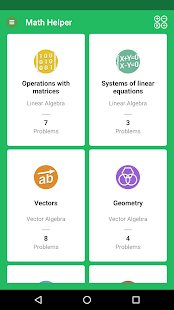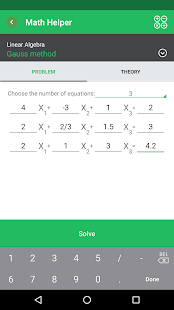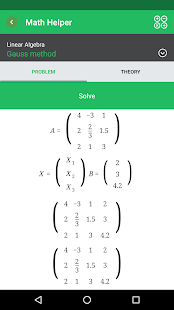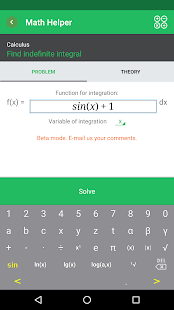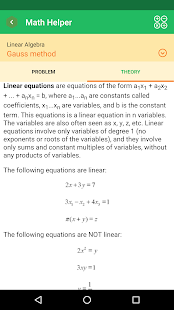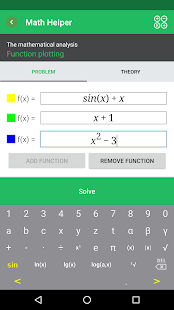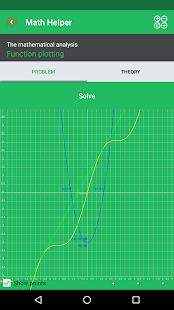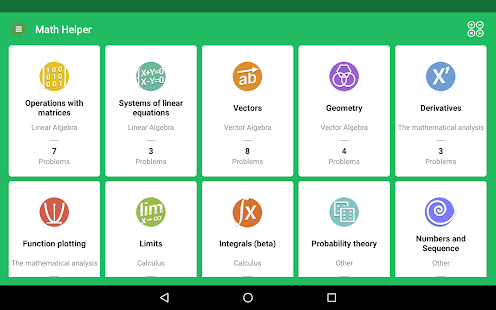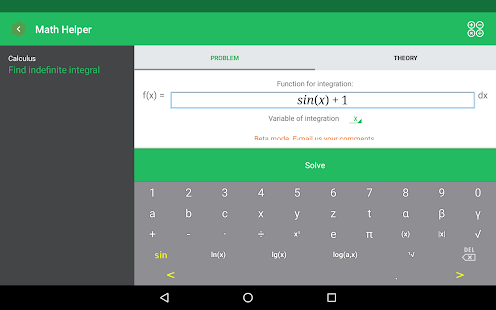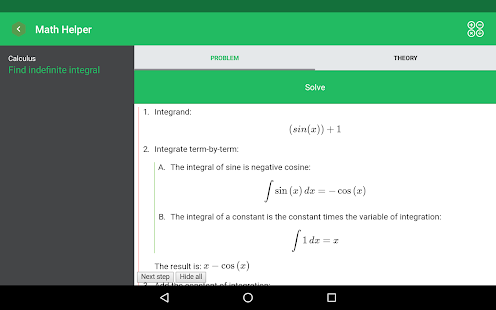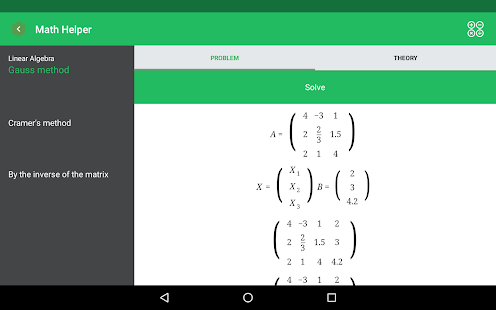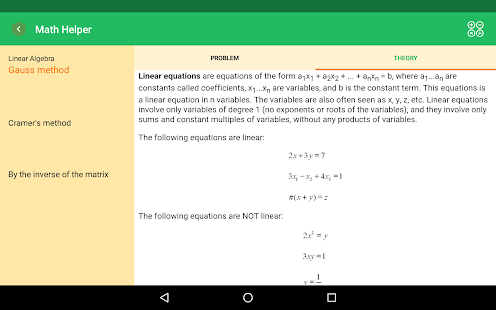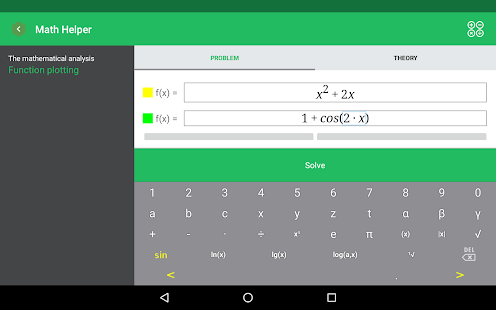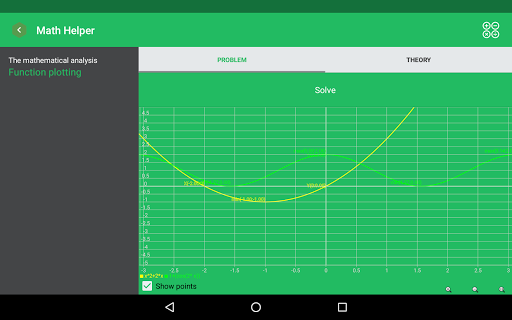Version 3.0.37 Uploader Hoster 200er uploaded.toDownload